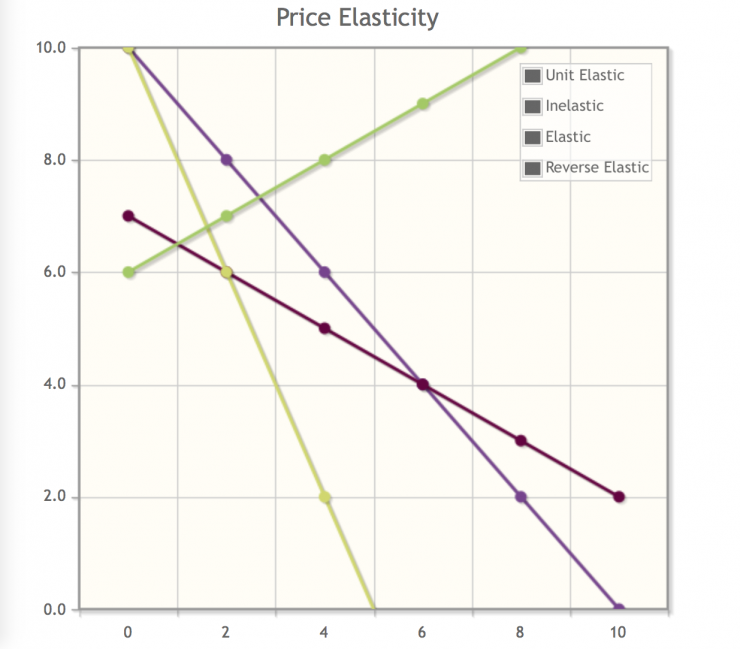# Price Elasticity (aka Price Elasticity of Demand)

If you do any reading or research about pricing you will come across the term “price elasticity”. Shortened from “price elasticity of demand” this is important (but easy) to understand.

Price elasticity is used to indicate how demand responds to a change in price. If you sell flowers and have ever wondered how your sales volume would be affected if you were to raise (or lower) your prices you are really wondering about the price elasticity of your product.

The precise measurement shows the percentage change in demand in response to a one percent change in price. It assumes that all other factors that affect demand, such as income, remain constant.

The number is arrived at by dividing the percentage change in demand by the percentage change in price. In the case of the flower shop let’s say that a 5% increase in prices led to a 10% decrease in demand. The formula would look like this…

#### Change in Demand ÷ Change in Price = PED (Price Elasticity) -10% ÷ 5% = -2

… meaning that your flowers have a price elasticity or PED of -2.

The law of demand states that, with all other factors being equal, an increase in price results in a decrease in demand. There are exceptions, like Veblen goods, but in most cases price elasticity will be negative.

Measurements of price elasticity are almost always negative, so much so that the negative sign is often excluded. For example the price elasticity of the sandwiches mentioned earlier is -2, but would often be discussed as simply “2”, omitting the negative because it can almost always be assumed.

### Elasticities of demand are interpreted as follows:

#### Perfectly Inelastic Demand

PED equals zero

When an increase in price does not lower demand at all price elasticity equals zero, also referred to as perfectly inelastic demand. If for example you could raise the price of your flowers without lowering demand at all it would be considered perfectly inelastic. Largely theoretical.

#### Inelastic or Relatively Inelastic Demand

PED greater than zero and less than one

When the decrease in demand is less than the increase in price it is referred to as inelastic or relatively inelastic demand. If for example you raised the prices of your flowers 10% and demand only decreased 5% you would have price elasticity of -0.5. Anything greater than -1 is considered inelastic or relatively inelastic demand.

#### Unit Elastic

PED equals one

When the increase in price equals the decrease in demand it is referred to as unit elastic. If for example a 5% increase in the price of your flowers resulted in a drop in demand of 5% it would be considered unit elastic.

#### Elastic or Relatively Elastic Demand

PED greater than zero

When the decrease in demand is larger than the increase in price it is referred to as elastic or relatively elastic demand. If for example a 10% increase in the price of your flowers results in a 25% decrease in demand (PED = -2.5) it is considered elastic or relatively elastic demand.

#### Perfectly Elastic Demand

PED equal infinity

When demand at a particular price is infinite and any increase in that price would decrease demand to zero it is said to be perfectly elastic. Like perfectly inelastic demand this is a largely theoretical situation

#### Reverse Elastic Demand

When demand increase alongside price it is called reverse elasticity. It seems strange but is commonly seen in luxury or Veblen goods.

For the most part perfect unit elasticity is theoretical. In the the real world demand is usually either relatively inelastic (the drop in demand is smaller than the the increase in price, meaning revenue goes up) or relatively elastic (the drop in demand is larger than the increase in price, meaning revenue goes down), and in both cases the "relatively" is usually dropped – we just refer to inelastic or elastic. There are also real world example of reverse elasticity, where demand rises alongside price.

### Examples

#### Inelastic

Generally speaking demand is considered inelastic (or relatively inelastic) when the PED is greater than negative one and changes in price have a relatively small effect on demand. Any reduction in demand will be offset by the increased price and overall revenue goes up.

One example would be fuel. Automobile fuel has a PED of approximately -0.09, meaning that a 1.0% increase in overall price (across a region) only reduces demand by less than 0.09%. Over the longer term people will start to reduce consumption by reducing travel, choosing more fuel efficient vehicles, etc. and the PED increases to -0.31, which is still relatively inelastic.

Cigarettes are another example with a PED that hovers around -0.4. Again increases in price do not significantly affect demand.

#### Elastic

Conversely demand is considered to be elastic (or relatively elastic) when its PED is less than negative one and changes in price have a relatively large effect on demand. Any increase in price will be offset by lowered demand and overall revenue goes down.

One example is recreational air travel, where a PED of -1.5 means that a 1% increase in price reduces demand by 1.5%. Liquor (PED -1.5) and brand name soda (PED 4.0) are also elastic as in each case reduced demand offsets any increase in price.

#### Reverse Elastic

There are (relatively few) very special cases that go against the law of demand – situations where increased prices also result in greater demand. These typically involve Veblen or Giffen goods and are covered separately.